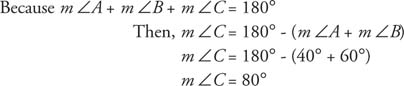## Angle Sum of a Triangle

With the use of the Parallel Postulate, the following theorem can be proven.

Theorem 25: The sum of the interior angles of any triangle is 180°.

m ∠ A + m ∠ B + m ∠ C = 180°.

Example 1: If m ∠ A = 40° and m ∠ B = 60°, find m ∠ C.